# The Basic Area Problem (introduction to the topic of integrals)

• I
Gold Member
TL;DR Summary
Trying to understand an introduction to the topic of the integral
Hi PF
There goes the quote:
The Basic Area Problem
In this section we are going to consider how to find the area of the region ##R## lying under the graph ##y=f(x)## of a nonnegative-valued, continous function ##f##, above the ##x##-axis and between the vertical lines ##x=a## and ##x=b##, where ##a<b##. (See Figure 5.4.) To accomplish this we proceed as follows. Divide the interval ##[a,b]## into ##n## subintervals by using division points:
$$a=x_0<x_1<x_2<x_3<\cdots<x_{n−1}<x_n=b$$
Denote by ##\Delta x_i## the length of the ##i##-th subinterval ##[x_{i−1},x_i]##:
$$\Delta x_i=x_i−x_{i−1},\qquad(i=1,2,3,...,n)$$
Vertically above each subinterval ##[x_{i−1},x_i]## build a rectangle whose base has length ##\Delta x_i## and whose height is ##f(x_i)##. The area of this rectangle is ##f(x_i)\Delta x_i##. Form the sum of these areas:
$$S_n=f(x_1)\Delta x_1+f(x_2)\Delta x_2+f(x_3)\Delta x_3+\cdots+f(x_n)\Delta x_n=\sum_{i=1}^n f(x_i)\Delta x_i$$
The rectangles are shown shaded in Figure 5.5 for a decreasing function ##f##. For an increasing function, the tops of the rectangles would lie above the graph of ##f## rather than below it. Evidently, ##S_n## is an approximation to the area of the region ##R##, and the approximation gets better as ##n## increases, provided we choose the points ##a=x_0<x_1<\cdots<x_n=b## in such a way that the width ##\Delta x_i## of the widest rectangle approaches zero.
Observe in Figure 5.6, for example, that subdividing a subinterval into two smaller subintervals reduces the error in the approximation by reducing that part of the area under the curve that is not contained in the rectangles. It is reasonable, therefore, to calculate the area ##R## by finding the limit of ##S_n## as ##n\rightarrow{\infty}## with the restriction that the largest of the subinterval widths ##\Delta x_i## must approach zero:
$$\displaystyle\text{Area of} R=\underset{\max\Delta x_i\to 0}{\lim_{n\to\infty}} S_n$$
Sometimes, but not always, it is useful to choose the points ##x_i (0\leq i\leq n)## in ##[a,b]## in such a way that the subinterval lengths ##\Delta x_i## are equal. In this case we have
$$\Delta x_i=\Delta x=\displaystyle\frac{b-a}{n},\qquad x_i=a+i\Delta x=a+\displaystyle\frac{i}{n}(b-a)$$

Doubt, question
Only one. It's clear that this is a good introduction. States two facts:
(i) the more we subdivide an interval, the better will be the approximation;
(ii) we have to take limits. Here comes the doubt: we must take limit of ##S_n## as ##n\rightarrow{\infty}## with the restriction that the largest of the subinterval widths ##\Delta x_i## must approach zero. It's ok, but, why must we pick the widest subinterval?

Attempt
No idea; my opinion is that choosing the most wide subinterval and taking limit to zero of it, this is, subdividing the most the largest one, we can forget about doing it to the whole interval... Is it understandable what I mean? I pray for it.

Greetings!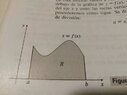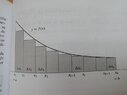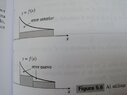Gold Member
TL;DR Summary: Trying to understand an introduction to the topic of the integral

(ii) we have to take limits. Here comes the doubt: we must take limit of Sn as n→∞ with the restriction that the largest of the subinterval widths Δxi must approach zero. It's ok, but, why must we pick the widest subinterval?
The widest subinterval width > any other subinterval width > 0
The widest goes to zero means all the subinterval widths go to zero together.

••hutchphd, Mark44 and mcastillo356
Mentor
To continue from what @anuttarasammyak said, if you had one subinterval that was, say, half the width of the whole interval, but you made all of the other subintervals smaller and smaller, that single large subinterval would prevent you from getting the true value of the area under the curve.
That's why it's necessary to stipulate that the largest of the subintervals must approach zero.

•mcastillo356 and Lnewqban
Gold Member
Hi PF, I will try to give an example: ##y=2+\cos x##. The plot is a decreasing function in the interval ##[a,b]=[0,\pi]##. Let's make a partition of this interval. The points would have a shape like ##a=x_0=0<x_1<x_2<x_3\cdots<x_{n-1}<\pi=x_n=b##. Let's see what happens: no matter how big is ##n##, I could make ##\{x_0,x_1,x_2,\cdots,x_{n-1}\}## points to be in a very short interval; say for example ##[0,\frac{\pi}{2}]##. But the way to avoid this is to make the biggest interval to tend to ##0##. This way we ensure to have points all the way long ##[a,b]##.
PD: this is not mine; I wouldn't have done without some guy's advice.
Greetings!

Mentor
Hi PF, I will try to give an example: ##y=2+\cos x##. The plot is a decreasing function in the interval ##[a,b]=[0,\pi]##. Let's make a partition of this interval. The points would have a shape like ##a=x_0=0<x_1<x_2<x_3\cdots<x_{n-1}<\pi=x_n=b##. Let's see what happens: no matter how big is ##n##, I could make ##\{x_0,x_1,x_2,\cdots,x_{n-1}\}## points to be in a very short interval; say for example ##[0,\frac{\pi}{2}]##. But the way to avoid this is to make the biggest interval to tend to ##0##. This way we ensure to have points all the way long ##[a,b]##.
This is pretty much what I said in post #3.

•mcastillo356
Gold Member
This is pretty much what I said in post #3.
Just the same, I would say. Now I realize. Is...It's the same thing. But I didn't see it.
Best whises, @Mark44 !

•hutchphd
Since you stipulated that your function should be continuous, the function will have a minimum and a maximum in every subinterval. Thus: Let mi be the minimum in interval i and let Mi be the maximum. Then $\sum_{i}m_{i}\Delta x_{i}\leq\sum_{i}f_{i}\Delta x_{i}\leq\sum_{i}M_{i}\Delta x_{i}$. This means that your estimated area will be limited above and below.
Now - what happens when you relax your condition a little bit and say "continuous, except for a single point"?

•mcastillo356
Gold Member
Now - what happens when you relax your condition a little bit and say "continuous, except for a single point"?
Good question. Continous in ##[0,\frac{\pi}{2})\cup(\frac{\pi}{2},\pi]##. Is this the suggested new condition? Intriguing indeed, in 48 hours I'll give a try.
Regards!

Mentor
Good question. Continous in ##[0,\frac{\pi}{2})\cup(\frac{\pi}{2},\pi]##. Is this the suggested new condition? Intriguing indeed, in 48 hours I'll give a try.
Here's an example of such a function: ##f(x) = \tan(x)## on ##[0, \pi/2)##...

•mcastillo356
Here's an example of such a function: ##f(x) = \tan(x)## on ##[0, \pi/2)##...
This is way beyond what I was thinking about. My first example was much more fundamental: $f(x)=1: x\in [0, \frac{\pi}{2}>\cup <\frac{\pi}{2}, \pi]; f(\frac{\pi}{2})=0$.
Another semi-example: $f(x)=$ (decimal part of x).

•mcastillo356
Mentor
Hi, something like this?

View attachment 321617

It's cosine function, ##x\in{[0,\frac{\pi}{2}})\cup{(\frac{\pi}{2},\pi]}##
No. Your function is defined on two disjoint intervals, but is also defined at the point you don't include. What @Svein and I had in mind were functions that were discontinuous at endpoints of their subdomains.

•mcastillo356
Gold Member
Since you stipulated that your function should be continuous, the function will have a minimum and a maximum in every subinterval. Thus: Let mi be the minimum in interval i and let Mi be the maximum. Then $\sum_{i}m_{i}\Delta x_{i}\leq\sum_{i}f_{i}\Delta x_{i}\leq\sum_{i}M_{i}\Delta x_{i}$. This means that your estimated area will be limited above and below.
Now - what happens when you relax your condition a little bit and say "continuous, except for a single point"?
Hello, dear PF, I lack the knowledge to face this exciting question for now. I have made the decision to focus on the textbook.

To continue from what @anuttarasammyak said, if you had one subinterval that was, say, half the width of the whole interval, but you made all of the other subintervals smaller and smaller, that single large subinterval would prevent you from getting the true value of the area under the curve.
That's why it's necessary to stipulate that the largest of the subintervals must approach zero.
Thanks!
P&L

Gold Member
Hi, @Svein, were you intruducing Riemann Sums and Partitions? I've turned the page of the textbook, and I have stumbled upon something very similar to your quote, and I sense that more powerful for the calculation of areas (see attached figure):

If ##A_i## is that part of the area under ##y=f(x)## and above the ##x##-axis that lies in the vertical strip between ##x=x_{i-1}## and ##x=x_i##, then
$$f(l_i)\Delta x_i\leq {A_i}\leq{f(u_i)\Delta x_i}$$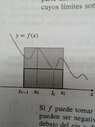Regards

Mentor
Hi, @Svein, were you intruducing Riemann Sums and Partitions? I've turned the page of the textbook, and I have stumbled upon something very similar to your quote, and I sense that more powerful for the calculation of areas (see attached figure):

If ##A_i## is that part of the area under ##y=f(x)## and above the ##x##-axis that lies in the vertical strip between ##x=x_{i-1}## and ##x=x_i##, then
$$f(l_i)\Delta x_i\leq {A_i}\leq{f(u_i)\Delta x_i}$$

View attachment 321784

Regards
If I can answer for Svein, that's the basic idea underlying the Riemann integral. In the limit as the number of subintervals increases without bound (again, assuming that the largest subinterval goes to zero), the lower Riemann sum and upper Riemann sum get closer together, trapping the value of the integral between them.

•mcastillo356
If I can answer for Svein, that's the basic idea underlying the Riemann integral. In the limit as the number of subintervals increases without bound (again, assuming that the largest subinterval goes to zero), the lower Riemann sum and upper Riemann sum get closer together, trapping the value of the integral between them.
Right on!

•mcastillo356
Gold Member
Hi,PF
If I can answer for Svein, that's the basic idea underlying the Riemann integral. In the limit as the number of subintervals increases without bound (again, assuming that the largest subinterval goes to zero), the lower Riemann sum and upper Riemann sum get closer together, trapping the value of the integral between them.

Upper and lower Riemann sums
The lower (Rieman) sum, L(f, P), and the upper (Riemann) sum, U(f, P), for the function ##f## and the partition ##P## are defined by:

$$L(f,P)=f(l_1)\Delta x_1+f(l_2)\Delta x_2+\cdots+f(l_n)\Delta x_n=\sum_{i=1}^n f(l_i)\Delta x_i$$

$$U(f,P)=f(u_1)\Delta x_1+f(u_2)\Delta x_2+\cdots+f(u_n)\Delta x_n=\sum_{i=1}^n f(u_i)\Delta x_i$$

EXAMPLE 2 Calculate the lower and upper Riemann sums for the function ##f(x)=x^2## on the interval ##[0,a]##, (where ##a>0##), corresponding to the partition ##P_n## of ##[0,a]## into ##n## subintervals of equal length.

$$L(f,P_n)=\frac{(n-1)(2n-1)a^3}{6n^2}$$

$$U(f,P_n)=\frac{(n-1)(2n-1)a^3}{6n^2}$$

(I've made a great argumentative leap)

EXAMPLE 3 Show that ##f(x)=x^2## is integrable over the interval ##[0,a]##, where ##a>0##, and evaluate ##\int_0^a x^2 \, dx##

We evaluate the limits as ##n\to \infty## of the lower and upper sums of ##f## over ##[0,a]## obtained in example 2 above

$$\lim_{n\to\infty}{L((f,P_n)}=\frac{a^3}{3}$$

$$\lim_{n\to\infty}{U((f,P_n)}=\frac{a^3}{3}$$

(Again, great ellipse)

If ##L(f,P_n)\leq I\leq {U(f,P_n)}##, we must have ##I=a^3/3##. Thus, ##f(x)=x^2## is integrable over ##[0,a]##, and

$$\int_0^a f(x)\, dx=\int_0^a x^2 \, dx=\frac{a^3}{3}$$

This is way beyond what I was thinking about. My first example was much more fundamental: $f(x)=1: x\in [0, \frac{\pi}{2}>\cup <\frac{\pi}{2}, \pi]; f(\frac{\pi}{2})=0$.
Is it anything like this image?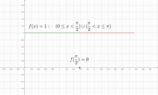I think this is an avoidable discontinuity, but the the textbook only devotes a small review to it

Gold Member
Hi, dear PF

I need some advice, or better said, opinions. One of the functions suggested on post #10 was

$$f(x)=\begin{cases}{1}&\text{if}& x\in [0,\pi]-\{\pi/2\}\\0 & \text{if}& x=\pi/2\end{cases}$$

Now comes the controversy: if it is an avoidable discontinuity, it becomes a rectangle; And I have calculated the are expeditously:

$$\mbox{Area}=\mbox{basis}\times{\mbox{height}}=\pi\times{1}=\pi\mbox{ squared units}$$

Avoiding overthinking, or avoiding the spirit of this thread, this is, Riemann?

Regards

If $\int_{0}^{\frac{\pi}{2}-\epsilon}f(x)dx$ exists for every ε and the limit $lim_{\epsilon \rightarrow 0} \int_{0}^{\frac{\pi}{2}-\epsilon}f(x)dx$ exists, then we define $\int_{0}^{\frac{\pi}{2}}f(x)dx$ to be that limit.

•mcastillo356
Gold Member
Hi, dear Forum, @Svein

If $\int_{0}^{\frac{\pi}{2}-\epsilon}f(x)dx$ exists for every ε and the limit $lim_{\epsilon \rightarrow 0} \int_{0}^{\frac{\pi}{2}-\epsilon}f(x)dx$ exists, then we define $\int_{0}^{\frac{\pi}{2}}f(x)dx$ to be that limit.

A quick search on the Internet has not given any clue. Could you give me some hint?

A quick search on the Internet has not given any clue. Could you give me some hint?
A long time ago, I could dig up a proof. Now I just have to cite from memory: "Functions that are continuous or piecewise continuous on an interval are Riemann integrable". Otherwise, I must refer to measure theory and talk about "sets of measure zero" (sets that can be covered by a collection af intervals with a total length of less than ε for any given ε) and that the integral af any bounded function over a set of measure zero is 0.
A set containing a single point has, of course a measure zero. Therefore, a finite number of singular points has also a measure zero.

•mcastillo356
Gold Member
"Functions that are continuous or piecewise continuous on an interval are Riemann integrable".
The last thing I just read in the texbook is similar to your quote: "If ##f## is continous on ##[a,b]##, then ##f## is integrable on ##[a,b]##". Two sentences later remarks: "We cannot, however, prove this theorem yet".
Conclusions: First, thank you; Second, I will continue reading the textbook, now like a donkey who has a carrot put in front of his eyesBest wishes!

Mentor
Now comes the controversy: if it is an avoidable discontinuity, it becomes a rectangle;
We usually say "removable discontinuity." With the function as you defined it, ##\int_0^{\pi} f(x)dx## does equal ##\pi##.
The other kind of discontinuity is exhibited with this function: ##f(x) = |\tan(x)|, x \in [0, \pi]##.

If $\int_{0}^{\frac{\pi}{2}-\epsilon}f(x)dx$ exists for every ε and the limit $lim_{\epsilon \rightarrow 0} \int_{0}^{\frac{\pi}{2}-\epsilon}f(x)dx$ exists, then we define $\int_{0}^{\frac{\pi}{2}}f(x)dx$ to be that limit.

A quick search on the Internet has not given any clue. Could you give me some hint?
You'll probably need to refer to a calculus textbook for a more in-depth explanation of what @Svein wrote.

As noted above, the function you wrote has a removable discontinuity:
$$f(x)=\begin{cases}{1}&\text{if}& x\in [0,\pi]-\{\pi/2\}\\0 & \text{if}& x=\pi/2\end{cases}$$
To evaluate the integral of this function you need to break up the interval into two improper integrals, and using limits at the discontinuity:
##\lim_{a \to \pi/2^-}\int_0^a 1 dx## and ##\lim_{b \to \pi/2^+}\int_b^\pi 1 dx##
Taking limits, we get ##\pi/2## for each integral, yielding ##\pi## for ##\int_0^\pi f(x)dx##.

In contrast, the function ##f(x) = |\tan(x)|## has a non-removable discontinuity in the interval ##[0, \pi]##. Using the technique I outlined above with improper integrals, neither integral converges to a finite number.

Gold Member
We usually say "removable discontinuity." With the function as you defined it, ##\int_0^{\pi} f(x)dx## does equal ##\pi##.
The other kind of discontinuity is exhibited with this function: ##f(x) = |\tan(x)|, x \in [0, \pi]##.
Nice.
You'll probably need to refer to a calculus textbook for a more in-depth explanation of what @Svein wrote.
Quite sure.
As noted above, the function you wrote has a removable discontinuity:
(...)
To evaluate the integral of this function you need to break up the interval into two improper integrals, and using limits at the discontinuity:
##\lim_{a \to \pi/2^-}\int_0^a 1 dx## and ##\lim_{b \to \pi/2^+}\int_b^\pi 1 dx##
Taking limits, we get ##\pi/2## for each integral, yielding ##\pi## for ##\int_0^\pi f(x)dx##
Not familiar to improper integrals. However, I understand what you explain.
In contrast, the function ##f(x) = |\tan(x)|## has a non-removable discontinuity in the interval ##[0, \pi]##. Using the technique I outlined above with improper integrals, neither integral converges to a finite number.
Fine. Nice again.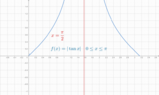Your post is exciting, comprehensible, and it suits my background perfectly. In essence, perfect for me, helpful.
Thanks!

Mentor
Not familiar to improper integrals.
They're dealt with in most calculus textbooks. Improper integrals typically are used when the integrand function isn't defined at one or both ends of the interval over which integration is being performed. Evaluating such an integral entails taking a limit, similar to what I did in my previous post.

•mcastillo356